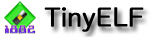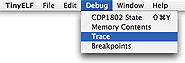## Using the Trace Panel

TinyELF allows you to view the last 1,000 instructions executed, along with comments revealing the effect of each instruction.To view the execution trace history, select the Trace command from TinyELF's Debug menu.The trace buffer can be cleared by clicking the Clear button.

Some of the comments may seem a little strange at first, because some instructions involve several values of interest. It helps to remember that the equals sign generally means that the value on the left side contains or points to the value on the right side. For instance, consider:

`ADD    .. D=1E, DF=0 from X=R4=00A5=06 + D=18`

The ADD instruction takes the memory byte pointed to by the register pointed to by X, adds it to the contents of D, and puts the carry flag in DF. Breaking down the comment above, we see:

• `D=1E, DF=0`
The result of the ADD is the value 1E in the D register, with a carry of 0 in DF.

• `from X=R4`
The explanation of how the result was arrived at begins here. We see that register X had been set to 4 at the time the ADD was executed.

• `=00A5`
Register 4 contained the value 00A5.

• `=06`
The memory location 00A5 contained the value 06. (Note, by the way, that all values are in hexadecimal.)

• `+ D=18`
Finally, this value, 06, was added to the contents of the D register, which was 18. 18 + 06 = 1E, with no carry.